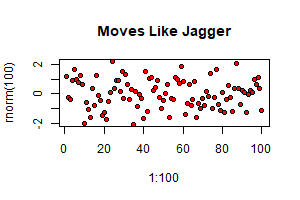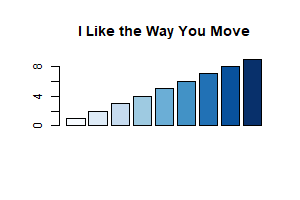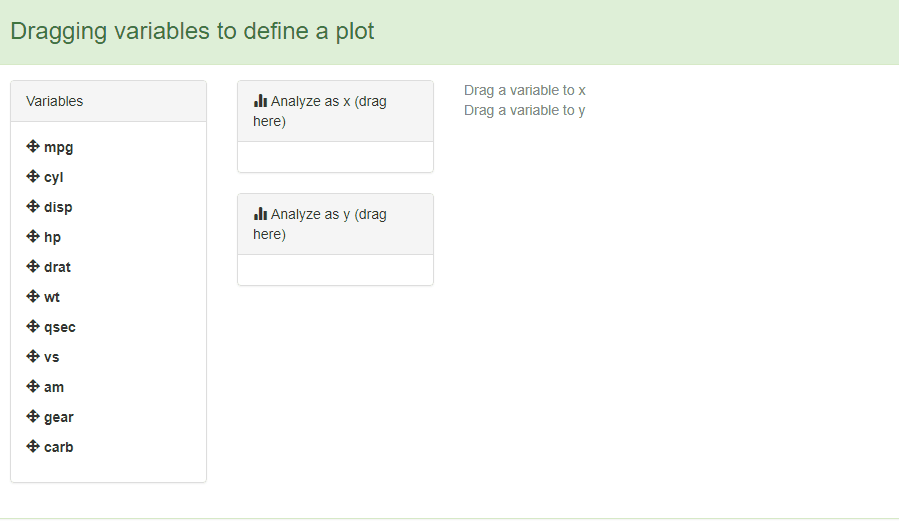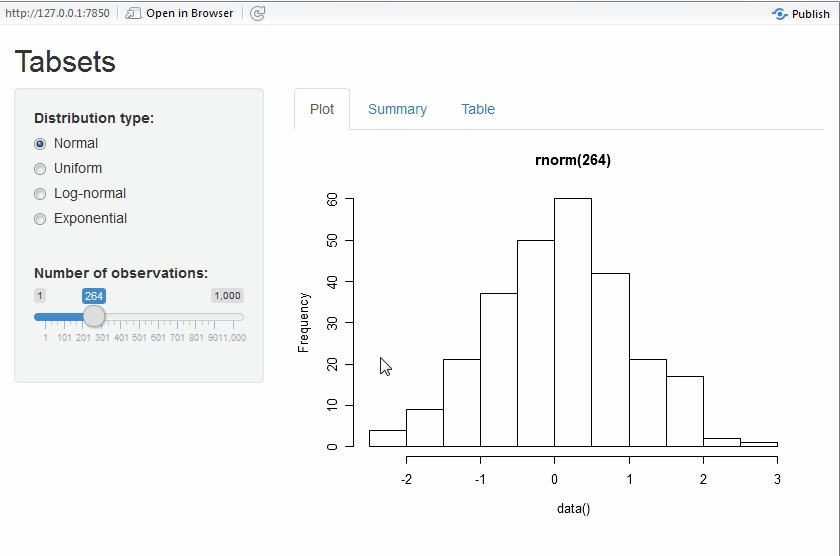# Understanding the interface to sortable.js

#### 2023-03-26

With the sortable htmlwidget you can use powerful, dependency-free interactivity from SortableJS in the browser, RStudio Viewer, or Shiny apps.

library(sortable)
library(htmltools)

## The central idea

The key idea to understand about sortable, and SortableJS in particular, is that the JavaScript will manipulate an HTML object based on it’s CSS id.

Using sortable in markdown is a little tricky since markdown does not provide an easy way to provide an id that we’ll need. We can overcome this by using bare HTML or using htmltools::tags. Let’s make a simple ul list. Note, however, that sortable works with nearly any HTML element, such as div.

### An example using raw HTML

The following example uses HTML to construct an unordered list (<ul>), and then uses sortable_js() to link the JavaScript required to create interactivity.

Note:

1. The HTML id matches the css_id argument of sortable_js().
2. You can drag and drop the list entries. Try it!
<p>You can drag and drop these items in any order (try it!):</p>
<ul id = "example01">
<li>Move</li>
<li>Or drag</li>
<li>Each of the items</li>
<li>To different positions</li>
</ul>
{r}
sortable_js(css_id = "example01")


You can drag and drop these items in any order (try it!):

• Move
• Or drag
• Each of the items
• To different positions

### Use a tag list to achieve the same, but from R

You can use the functions tags() and tagList(), both from the htmltools package, to create HTML.

This means you can construct the sortable list using:

library(htmltools)
tagList(
tags$ul( id = "example02", tags$li("drag me"),
tags$li("sort me"), tags$li("any way you like")
),
sortable_js("example02")
)
• drag me
• sort me
• any way you like

### Little harder but better example

The SortableJS functionality works with any HTML object, not just lists.

In this next example, you can see how to drag and drop images (<img>). To embed the plots on the page, you can use the base64::img() function to encode the png images into a format that HTML understands.

library(base64enc)
library(withr)

# create two plots for demo purposes
pngfile_1 <- tempfile(fileext = ".png")
with_png(pngfile_1, width = 300, height = 200,{
plot(1:100, rnorm(100), pch = 21, bg = "red")
title(main = "Moves Like Jagger")
})

pngfile_2 <- tempfile(fileext = ".png")
with_png(pngfile_2, width = 300, height = 200,{
barplot(1:9, col = blues9)
title(main = "I Like the Way You Move")
})

Again, notice that the HTML id matches the css_id.

tagList(
tags$div( id = "example03", tags$image(src = base64enc::dataURI(file = pngfile_1, mime = "image/png")),
tags$image(src = base64enc::dataURI(file = pngfile_2, mime = "image/png")) ), sortable_js(css_id = "example03") )## The power of groups Looking at the SortableJS excites me about the potential to use sortable as an important UI element in both a Shiny and non-Shiny context. We could potentially demo a plot builder with something like this example. You’ll notice that it doesn’t really do anything, but I hope the intent and direction is clear. knitr::read_chunk( system.file("shiny-examples/drag_vars_to_plot/app.R", package = "sortable") )## Dragging and dropping shiny tabs The sortable JS library allows movable tabs inside a Shiny (and also not Shiny) app. By adding just one line of code and an id to this RStudio Tabset example, you get tabs that the user can re-arrange. You can copy and paste to see it for yourself, or runGist("2dbe45f77b65e28acab9"). The modified code snippet is: # Show a tabset that includes a plot, summary, and table view # of the generated distribution mainPanel( tabsetPanel( type = "tabs", id = "sortTab", tabPanel("Plot", plotOutput("plot")), tabPanel("Summary", verbatimTextOutput("summary")), tabPanel("Table", tableOutput("table")) ) ) ), sortable_js("sortTab") And the full code: ## Example shiny app to drag-and-drop tabsets in a shiny app # all credit for code goes to RStudio # https://github.com/rstudio/shiny-examples/tree/main/006-tabsets library(sortable) library(shiny) ui = # Define UI for random distribution application shinyUI(fluidPage( # Application title titlePanel("Tabsets"), # Sidebar with controls to select the random distribution type # and number of observations to generate. Note the use of the # br() element to introduce extra vertical spacing sidebarLayout( sidebarPanel( radioButtons( "dist", "Distribution type:", c( "Normal" = "norm", "Uniform" = "unif", "Log-normal" = "lnorm", "Exponential" = "exp" ) ), br(), sliderInput( "n", "Number of observations:", value = 500, min = 1, max = 1000) ), # Show a tabset that includes a plot, summary, and table view # of the generated distribution mainPanel( tabsetPanel( type = "tabs", id = "sortTab", tabPanel("Plot", plotOutput("plot")), tabPanel("Summary", verbatimTextOutput("summary")), tabPanel("Table", tableOutput("table")) ) ) ), sortable_js("sortTab") )) server = function(input, output) { # Reactive expression to generate the requested distribution. # This is called whenever the inputs change. The output # functions defined below then all use the value computed from # this expression data <- reactive({ dist <- switch( input$dist,
norm = rnorm,
unif = runif,
lnorm = rlnorm,
exp = rexp,
rnorm
)

dist(input$n) }) # Generate a plot of the data. Also uses the inputs to build # the plot label. Note that the dependencies on both the inputs # and the data reactive expression are both tracked, and # all expressions are called in the sequence implied by the # dependency graph output$plot <- renderPlot({
dist <- input$dist n <- input$n

hist(data(),
main=paste('r', dist, '(', n, ')', sep=''))
})

# Generate a summary of the data
output$summary <- renderPrint({ summary(data()) }) # Generate an HTML table view of the data output$table <- renderTable({
data.frame(x = data())
})

}

shinyApp( ui, server )